Numerical Steepest Descent
(pain-free numerical integration of oscillatory analytic functions)
Alex Barnett 10/2/18, notes made with Matlab Live Editor outputted to HTML.
See handwritten notes for theory.
A) Warm up with "infinite" trapezoid rule applied to the Gaussian:
f = @(x) exp(-x.^2/2); % std Gaussian
I = sqrt(2*pi); % exact integral
hs = 0.6:.01:2;
Es = nan*hs;
for i=1:numel(hs), h=hs(i);
Es(i) = abs(I - h*sum(f(h*(-100:100)))); % enough points to get tail
end
figure; semilogy(hs,Es,'.-'); hold on; plot(hs,5*exp(-20./hs.^2),'r-');
xlabel('h'); ylabel('E_h'); title('Gaussian integral via inf trap rule')
legend('inf trap rule','5e^{-20/h^2}')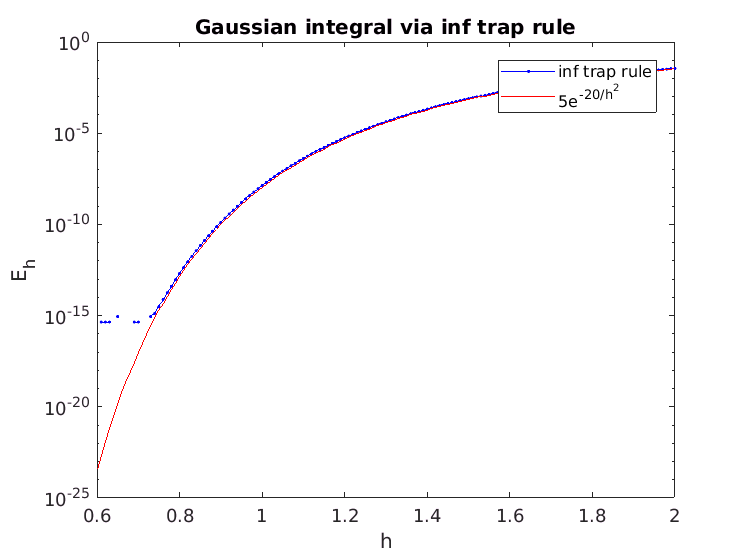Note how well the predicted error matches the actual quadrature error convergence, down to machine precision.
h=0.8 gets 13-digit accuracy, and since the integrand decays, eg f(8) ~ 1e-14, then only around 20 nodes are needed in the sum:
% check truncated trap rule (no end-point corrections or Legendre), rel err:
h = .8; xj = h*(-10:10); abs(I - h*sum(f(xj))) / I
ans = 8.09648446702193e-14
figure; t = -10:.01:10; plot(t,f(t),'-');
hold on; plot(xj,f(xj),'k.','markersize',10);
title('Gaussian: 21 quadr pts, 13 digits')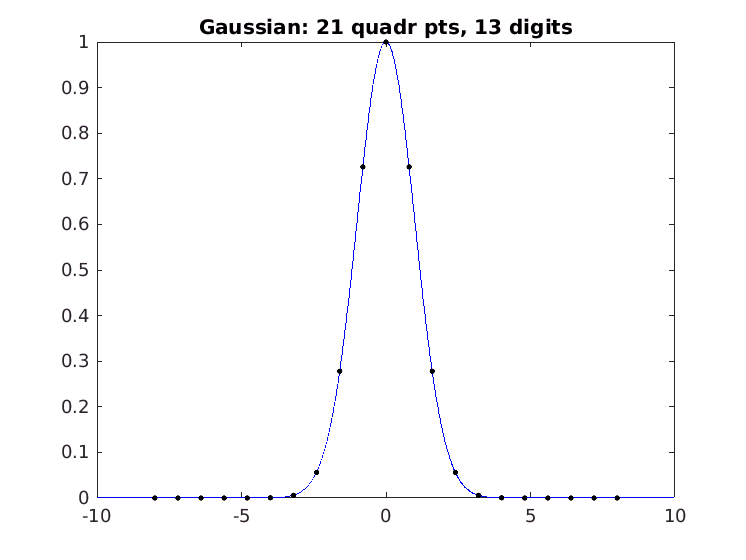In case you're wondering, this particular fractional offset of the grid is not special:
% arbitrary fractional offset of grid matters? no:
xj = xj+0.3; abs(I - h*sum(f(xj))) / I
ans = 5.70474397895199e-14
The Gaussian looks much more scary in the complex plane:
% what looks like in complex plane?
figure; [xx yy] = meshgrid(t,t); zz = xx+1i*yy;
imagesc(t,t,real(f(zz))); caxis(3*[-1 1]); axis xy equal tight;
xlabel('Re z'); ylabel('Im z'); title('Re Gaussian in complex plane')
colorbar; hold on; plot(xj,0*xj,'k.','markersize',10); % nodes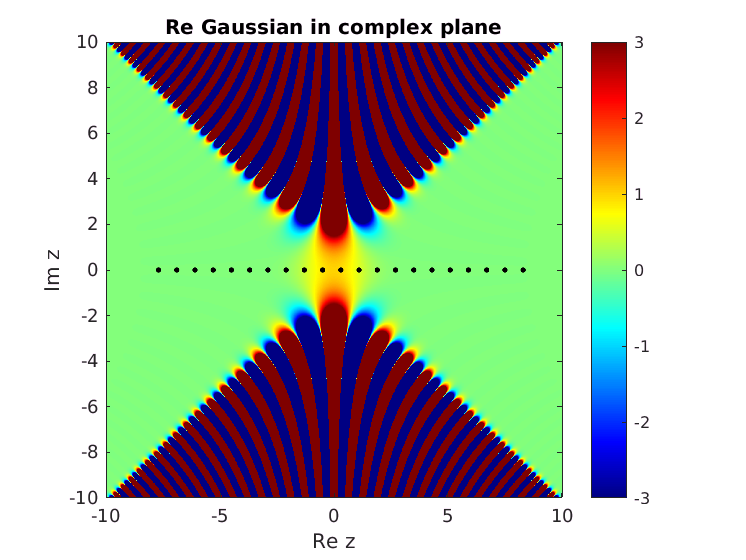Note the exponentially large oscillations in the regions within 45 degrees of the Im axis.
B) We now apply such integration to a related integral that is instead oscillatory.
Imagine g(x) = (1/(1+x^2)) * cos(30*(x-1)^2)
We note it is the Re part of the following analytic function, which we notice is highly oscillatory, so would be very slow to use adaptive integration on. We examine in the complex plane:
f = @(z) (1./(1+z.^2)) .* exp(1i*30*(z-1).^2);
gx = -2:1e-3:3; figure; plot(gx,real(f(gx)),'-');
title('oscillatory real analytic func g');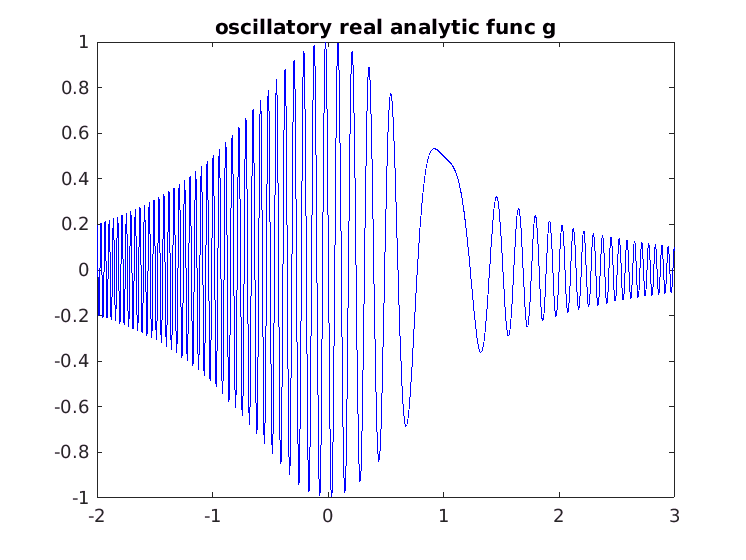gy = -2:1e-3:2; [xx yy] = meshgrid(gx,gy); zz = xx+1i*yy; fz = f(zz);
figure; imagesc(gx,gy,real(fz)); caxis(3*[-1 1]); axis xy equal tight;
xlabel('Re z'); ylabel('Im z'); title('Re f(z) in complex plane')
Noting the function f(z) has the form a(z) * exp( i b(z) ) where a is the slowly-varying amplitude function, and b(z) is the slowly-varying phase function, we look for "stationary phase" points x_0 on the real axis where b'(x_0) = 0. There is only one. We also note that b''(x_0) = 60, and that the local behavior is that of the Gaussian rotated by 45 degrees. The spatial rescaling of our quadrature scheme is thus by sqrt(60), and rotation by 45 degrees (although it does not have to be exactly this):
x0 = 1; % stationary phase pt where b'(x0) = 0
w = exp(1i*pi/4)/sqrt(60); % rot & rescale
We check convergence of a 21-point quadrature rule vs h scaled around this point, and easily get 12 digit accuracy:
for h=1.0:-0.1:0.7 % converge test: spacings for std real Gaussian
zj = x0 + w*h*(-10:10); % center around stat phase, scale by w
disp([h real(w*h*sum(f(zj)))])
end
1 0.113480873126374
0.9 0.113480872253493
0.8 0.113480872243906
0.7 0.113480872243883
One should also check it's converged with respect to the number of points:
zj = x0 + w*h*(-12:12); % try a few more points
disp([h real(w*h*sum(f(zj)))])
0.7 0.113480872243889
And the result is independent of small changes in angle:
w = exp(1.1*1i*pi/4)/sqrt(60); % rot & rescale
zj = x0 + w*h*(-10:10); % center around stat phase, scale by w
disp([h real(w*h*sum(f(zj)))])
0.7 0.113480872243912
Finally we add the points to the plot. Note that actually there are poles in a(z) at +-i but these are either weak or far from the integration contour, so don't reduce the convergence rate:
hold on; plot(zj,'k.','markersize',10)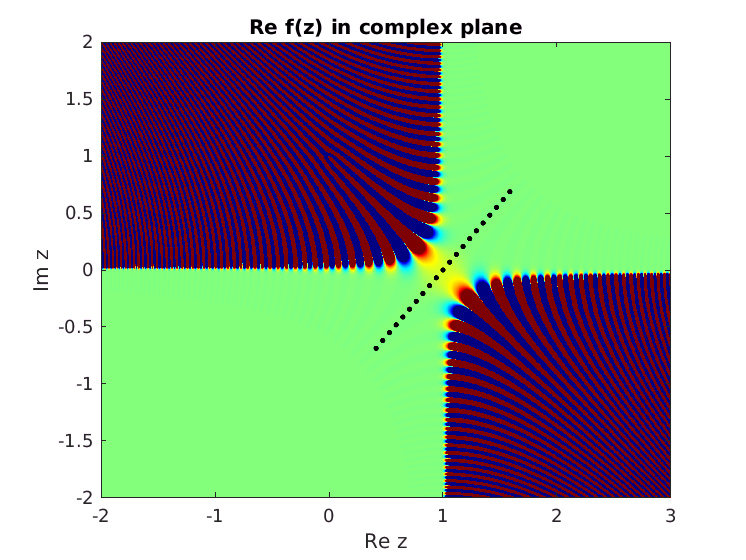One can also use smooth analytic curves to map the periodic trapezoid rule, and to pass through multiple stationary phase points.
Note how the above differs from the analytic "method of steepest descent" which merly gives asymptotics in the large parameter in b(z).
Also note that other researchers, such as Daan Huybrechs, have related "numerical steepest descent" methods. However the above is about as simple as its gets.

## C) References:

The SIAM 100-Digit Challenge: A Study in High-Accuracy Numerical Computing, Folkmar Bornemann · Dirk Laurie · Stan Wagon · Jörg Waldvogel (SIAM, 2004).
"High-order boundary integral equation solution of high frequency wave scattering from obstacles in an unbounded linearly stratified medium", A. H. Barnett, B. J. Nelson, and M. Mahoney, J. Comput. Phys. 297, 407-426 (2015).
F. W. J. Olver, Asymptotics and Special Functions (Academic Press, 1974).